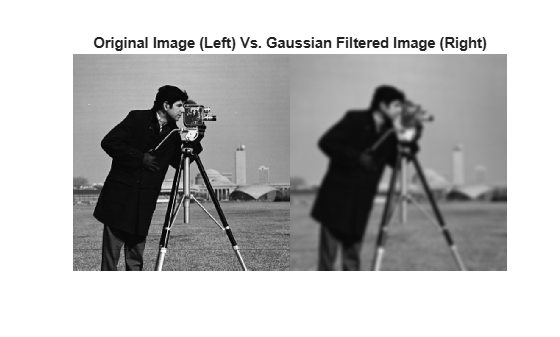# imgaussfilt

2-D Gaussian filtering of images

## Syntax

``B = imgaussfilt(A)``
``B = imgaussfilt(A,sigma)``
``B = imgaussfilt(___,Name,Value)``

## Description

````B = imgaussfilt(A)` filters image `A` with a 2-D Gaussian smoothing kernel with standard deviation of 0.5, and returns the filtered image in `B`.```

example

````B = imgaussfilt(A,sigma)` filters image `A` with a 2-D Gaussian smoothing kernel with standard deviation specified by `sigma`.```
````B = imgaussfilt(___,Name,Value)` uses name-value arguments to control aspects of the filtering.```

## Examples

collapse all

`I = imread('cameraman.tif');`

Filter the image with a Gaussian filter with standard deviation of 2.

`Iblur = imgaussfilt(I,2);`

Display the original and filtered image in a montage.

```montage({I,Iblur}) title('Original Image (Left) Vs. Gaussian Filtered Image (Right)')```## Input Arguments

collapse all

Image to be filtered, specified as a numeric array of any dimension.

Data Types: `single` | `double` | `int8` | `int16` | `int32` | `uint8` | `uint16` | `uint32`

Standard deviation of the Gaussian distribution, specified as a positive number or a 2-element vector of positive numbers. If you specify a scalar, then `imgaussfilt` uses a square Gaussian kernel.

Data Types: `single` | `double` | `int8` | `int16` | `int32` | `int64` | `uint8` | `uint16` | `uint32` | `uint64`

### Name-Value Arguments

Specify optional pairs of arguments as `Name1=Value1,...,NameN=ValueN`, where `Name` is the argument name and `Value` is the corresponding value. Name-value arguments must appear after other arguments, but the order of the pairs does not matter.

Before R2021a, use commas to separate each name and value, and enclose `Name` in quotes.

Example: `'FilterSize',3`

Size of the Gaussian filter, specified as a positive, odd integer or 2-element vector of positive, odd integers. If you specify a scalar, then `imgaussfilt` uses a square filter. The default filter size is `2*ceil(2*sigma)+1`.

Data Types: `single` | `double` | `int8` | `int16` | `int32` | `int64` | `uint8` | `uint16` | `uint32` | `uint64`

Image padding, specified as one of the following.

ValueDescription
numeric scalarPad image with elements of constant value.
`'circular'`

Pad with circular repetition of elements within the dimension.

`'replicate'`

Pad by repeating border elements of array.

`'symmetric'`

Pad image with mirror reflections of itself.

Data Types: `single` | `double` | `int8` | `int16` | `int32` | `int64` | `uint8` | `uint16` | `uint32` | `uint64` | `logical` | `char` | `string`

Domain in which to perform filtering, specified as one of the following values:

ValueDescription
`'auto'`Perform convolution in the spatial or frequency domain, based on internal heuristics.
`'frequency'`Perform convolution in the frequency domain.
`'spatial'`Perform convolution in the spatial domain.

Data Types: `char` | `string`

## Output Arguments

collapse all

Filtered image, returned as a numeric array of the same class and size as the input image, `A`.

## Tips

• If image `A` contains elements with values `Inf` or `NaN`, then the behavior of `imgaussfilt` for frequency domain filtering is undefined. This can happen if you set the `'FilterDomain'` name-value argument to `'frequency'` or if you set it to `'auto'` and `imgaussfilt` uses frequency domain filtering. To restrict the propagation of `Inf`s and `NaN`s in the output in a manner similar to `imfilter`, consider setting the `'FilterDomain'` name-value argument to `'spatial'`.

• If you set the `'FilterDomain'` name-value argument to `'auto'`, then `imgaussfilt` uses an internal heuristic to determine whether spatial or frequency domain filtering is faster. This heuristic is machine dependent and may vary for different configurations. For optimal performance, try both options, `'spatial'` and `'frequency'`, to determine the best filtering domain for your image and kernel size.

• If you do not specify the `'Padding'` name-value argument, then `imgaussfilt` uses `'replicate'` padding by default, which is different from the default used by `imfilter`.

## Version History

Introduced in R2015a